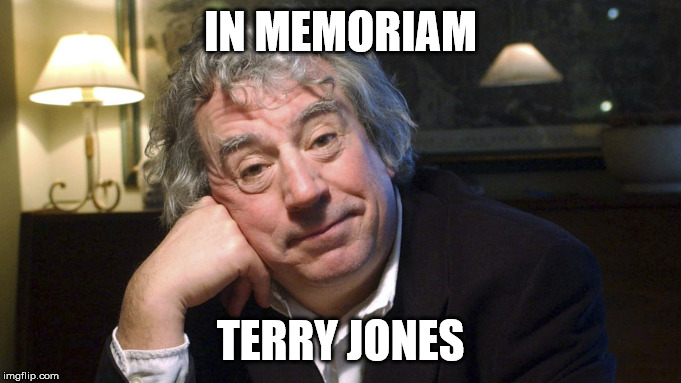-=+=- -=+=- -=+=- -=+=- -=+=- -=+=- -=+=- -=+=- -=+=- -=+=- -=+=- -=+=- -=+=- -=+=- -=+=- -=+=- -=+=- -=+=- -=+=- -=+=- -=+=- -=+=- -=+=- -=+=- -=+=- -=+=- -=+=- -=+=- -=+=- -=+=- (c) WidthPadding Industries 1987 0|673|0 -=+=- -=+=- -=+=- -=+=- -=+=- -=+=- -=+=- -=+=- -=+=- -=+=- -=+=- -=+=- -=+=- -=+=- -=+=- -=+=- -=+=- -=+=- -=+=- -=+=- -=+=- -=+=- -=+=- -=+=- -=+=- -=+=- -=+=- -=+=- -=+=- -=+=- Socoder -> Off Topic -> RIP : Terry Jones Posted : Wednesday, 22 January 2020, 12:43 JayenkaiRIP : Terry Jones Linkage -=-=- ''Load, Next List!''Posted : Wednesday, 22 January 2020, 12:43 rockfordR.I.P. Posted : Wednesday, 22 January 2020, 12:48 Jayenkai-=-=- ''Load, Next List!''Posted : Wednesday, 22 January 2020, 14:19 Tricky"Two down, four to go"... I guess, it's a typical thing for Cleese to say... (Well, Terry was the second to pass, as Graham Chapman was the first). I am a bit sad.... Monty Python was a great inspiration for me... Heck, they even helped me to find myself as a writer (not in the sense that I met them, but you know what I mean, right?)